• 在进行t检验、F检验之前，我们往往要求样本大致服从正态分布，下面介绍两种检验样本是否服从正态分布的方法。 1 可视化 我们可以通过将样本可视化，看一下样本的概率密度是否是正态分布来初步判断样本是否服从正态...
在进行t检验、F检验之前，我们往往要求样本大致服从正态分布，下面介绍两种检验样本是否服从正态分布的方法。

1 可视化

我们可以通过将样本可视化，看一下样本的概率密度是否是正态分布来初步判断样本是否服从正态分布。

代码如下：

import numpy as np
import pandas as pd
import matplotlib.pyplot as plt

# 使用pandas和numpy生成一组仿真数据
s = pd.DataFrame(np.random.randn(500),columns=['value'])
print(s.shape)      # (500, 1)

# 创建自定义图像
fig = plt.figure(figsize=(10, 6))
# 创建子图1
ax1 = fig.add_subplot(2,1,1)
# 绘制散点图
ax1.scatter(s.index, s.values)
plt.grid()      # 添加网格

# 创建子图2
ax2 = fig.add_subplot(2, 1, 2)
# 绘制直方图
s.hist(bins=30,alpha=0.5,ax=ax2)
# 绘制密度图
s.plot(kind='kde', secondary_y=True,ax=ax2)     # 使用双坐标轴
plt.grid()      # 添加网格

# 显示自定义图像
plt.show()

可视化图像如下：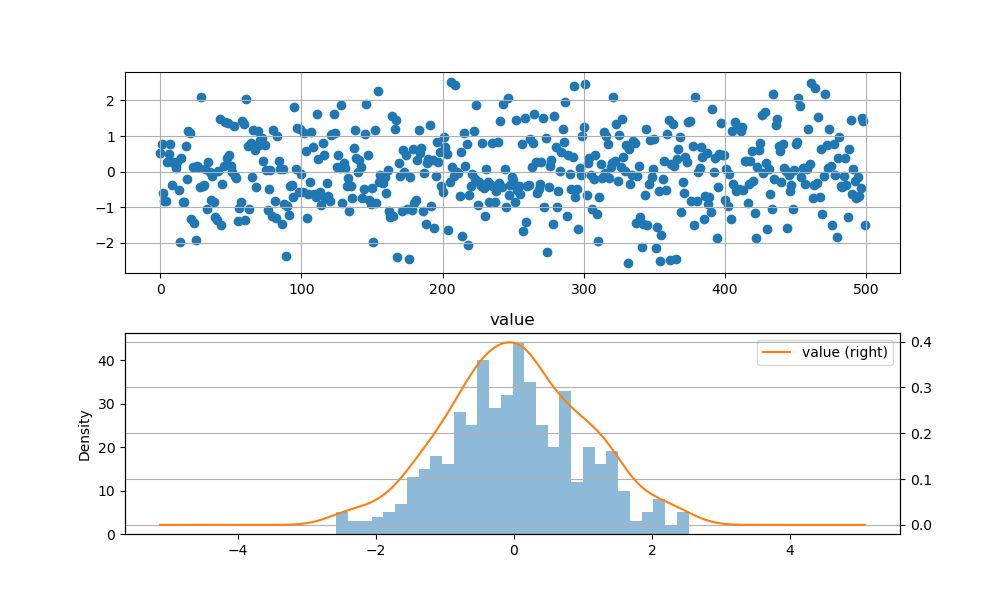从图中可以初步看出生成的数据近似服从正态分布。为了得到更具说服力的结果，我们可以使用统计检验的方法，这里使用的是.scipy.stats中的函数。

2 统计检验

1）kstest

scipy.stats.kstest函数可用于检验样本是否服从正态、指数、伽马等分布，函数的源代码为：

def kstest(rvs, cdf, args=(), N=20, alternative='two-sided', mode='approx'):
"""
Perform the Kolmogorov-Smirnov test for goodness of fit.

This performs a test of the distribution F(x) of an observed
random variable against a given distribution G(x). Under the null
hypothesis the two distributions are identical, F(x)=G(x). The
alternative hypothesis can be either 'two-sided' (default), 'less'
or 'greater'. The KS test is only valid for continuous distributions.

Parameters
----------
rvs : str, array or callable
If a string, it should be the name of a distribution in scipy.stats.
If an array, it should be a 1-D array of observations of random
variables.
If a callable, it should be a function to generate random variables;
it is required to have a keyword argument size.
cdf : str or callable
If a string, it should be the name of a distribution in scipy.stats.
If rvs is a string then cdf can be False or the same as rvs.
If a callable, that callable is used to calculate the cdf.
args : tuple, sequence, optional
Distribution parameters, used if rvs or cdf are strings.
N : int, optional
Sample size if rvs is string or callable.  Default is 20.
alternative : {'two-sided', 'less','greater'}, optional
Defines the alternative hypothesis (see explanation above).
Default is 'two-sided'.
mode : 'approx' (default) or 'asymp', optional
Defines the distribution used for calculating the p-value.

- 'approx' : use approximation to exact distribution of test statistic
- 'asymp' : use asymptotic distribution of test statistic

Returns
-------
statistic : float
KS test statistic, either D, D+ or D-.
pvalue :  float
One-tailed or two-tailed p-value.

2）normaltest

scipy.stats.normaltest函数专门用于检验样本是否服从正态分布，函数的源代码为：

def normaltest(a, axis=0, nan_policy='propagate'):
"""
Test whether a sample differs from a normal distribution.

This function tests the null hypothesis that a sample comes
from a normal distribution.  It is based on D'Agostino and
Pearson's _, _ test that combines skew and kurtosis to
produce an omnibus test of normality.

Parameters
----------
a : array_like
The array containing the sample to be tested.
axis : int or None, optional
Axis along which to compute test. Default is 0. If None,
compute over the whole array a.
nan_policy : {'propagate', 'raise', 'omit'}, optional
Defines how to handle when input contains nan. 'propagate' returns nan,
'raise' throws an error, 'omit' performs the calculations ignoring nan
values. Default is 'propagate'.

Returns
-------
statistic : float or array
s^2 + k^2, where s is the z-score returned by skewtest and
k is the z-score returned by kurtosistest.
pvalue : float or array
A 2-sided chi squared probability for the hypothesis test.

3）shapiro

scipy.stats.shapiro函数也是用于专门做正态检验的，函数的源代码为：

def shapiro(x):
"""
Perform the Shapiro-Wilk test for normality.

The Shapiro-Wilk test tests the null hypothesis that the
data was drawn from a normal distribution.

Parameters
----------
x : array_like
Array of sample data.

Returns
-------
W : float
The test statistic.
p-value : float
The p-value for the hypothesis test.

下面我们使用第一部分生成的仿真数据，用这三种统计检验函数检验生成的样本是否服从正态分布（p > 0.05），代码如下：

import numpy as np
import pandas as pd
import matplotlib.pyplot as plt

# 使用pandas和numpy生成一组仿真数据
s = pd.DataFrame(np.random.randn(500),columns=['value'])
print(s.shape)      # (500, 1)

# 计算均值
u = s['value'].mean()
# 计算标准差
std = s['value'].std()  # 计算标准差
print('scipy.stats.kstest统计检验结果：----------------------------------------------------')
print(stats.kstest(s['value'], 'norm', (u, std)))
print('scipy.stats.normaltest统计检验结果：----------------------------------------------------')
print(stats.normaltest(s['value']))
print('scipy.stats.shapiro统计检验结果：----------------------------------------------------')
print(stats.shapiro(s['value']))

统计检验结果如下：

scipy.stats.kstest统计检验结果：----------------------------------------------------
KstestResult(statistic=0.01596290473494305, pvalue=0.9995623150120069)
scipy.stats.normaltest统计检验结果：----------------------------------------------------
NormaltestResult(statistic=0.5561685865675511, pvalue=0.7572329891688141)
scipy.stats.shapiro统计检验结果：----------------------------------------------------
(0.9985257983207703, 0.9540967345237732)

可以看到使用三种方法检验样本是否服从正态分布的结果中p-value都大于0.05，说明服从原假设，即生成的仿真数据服从正态分布。

参考

python数据分析----卡方检验，T检验，F检验，K-S检验

python使用scipy.stats数据（正态）分布检验方法

python 如何判断一组数据是否符合正态分布

展开全文python 数据分析
• 如何检验样本是否服从正态分布？ 可以使用Q-Q图来进行检验，Q-Q图是一个散点图，点(x, y)表示数据x的某个分位数，y表示和x的分位数相同的分位数（即FX(x)=FY(y)F_X (x)=F_Y(y)），如果说两个分布的QQ图在一条直线上...
在数据分析中如果某个数据服从正态分布的话，我们可以利用正态分布的性质做出很多有意义的分析，例如t-检验。。

如何检验样本是否服从正态分布？
可以使用Q-Q图来进行检验，Q-Q图是一个散点图，点(x, y)表示数据x的某个分位数，y表示和x的分位数相同的分位数（即FX(x)=FY(y)<!--//--><![CDATA[//><!--
F_X (x)=F_Y(y)
//--><!]]>），如果说两个分布的QQ图在一条直线上，则说明每个[xi,xi+1]，[yi,yi+1]<!--//--><![CDATA[//><!--
[x_i, x_{i+1}]，[y_i, y_{i+1}]
//--><!]]>区间所包含的数据在整个数据集中的比例相同，也就是说明如果对x或y进行放缩的话可以让它们。

所以，如果把未知数据和标准正态分布做Q-Q图的话，如果所有点在一条直线上则说明未知数据的分布服从正态分布。

如果不是正态分布怎么办？
数据右偏的话可以对所有数据取对数、取平方根等，它的原理是因为这样的变换的导数是逐渐减小的，也就是说它的增速逐渐减缓，所以就可以把大的数据向左移，使数据接近正态分布。
如果左偏的话可以取相反数转化为右偏的情况。
展开全文• 在得到一系列数据后，如何利用SPSS去检测这些数据分布是否服从正态分布
• 而今天学习的jbtest()函数就可以判断数据是否服从正态分布 Jarque-Bera检验简单理论 在统计学中，Jarque-Bera检验是对样本数据是否具有符合正态分布的偏度和峰度的拟合优度的检验，其统计测试结果总是非负的。其结果...
在搞数学建模中，一直流传着这样一句话，数模数模，学到就是赚到。每一项技术都可以在现实生活中得到广泛的应用。就犹如正态分布跟二八法则一样。而今天学习的jbtest()函数就可以判断数据是否服从正态分布
Jarque-Bera检验简单理论
在统计学中，Jarque-Bera检验是对样本数据是否具有符合正态分布的偏度和峰度的拟合优度的检验，其统计测试结果总是非负的。其结果远大于0，则表示数据不具有正态分布。
正态分布概率纸
正态概率纸是一张特殊刻度的坐标纸，使得对于任何正态分布，将(x,F(x))在该坐标纸上点图，则成一条直线。正态分布不同，相应的直线不同
正态概率纸的用处很多：最简单的就是查正态分布函数，检验分布的正态性和估计正态分布的参数μ和sigama
例子：淮河流域历史上:经常发生洪水灾害，据统计1949~1991年淮河流域成灾面积(单位:万亩)每年总计分别为下列
>> x = [3383.4 4687.4 1631.1 2244.5 2011.7 6123.1 1918.0 6232.4 ...
5453.9 1412.4 321.5 2185.0 1285.4 4079.6 10124.2 5532.7 ...
3809.3 389.4 412.1 809.7 870.6 1055.7 1451.8 1532.9 765.9 ...
1987.6 2765.5 739.9 515.6 428.4 3794.5 242.3 4812 2204.7 ...
4407.1 2885 1124.7 1190 191.4 2227.9 2079 6934.1];

实验步骤

数据录入进matlab里
使用normplot(x) 概率值验证是否服从正态分布
采用jbtest(x,0.05) 正态分布的拟合的检验

normplot函数与jbtest函数使用
前者只需要将x的随机数据传入进去比如
x= [1 2 3 4]
normplot(x)

第二个函数用来对正态分布函数进行拟合检验，第一个参数也是x数据，第二参数就是置信水平α默认0.05
实验代码


>> x = [3383.4 4687.4 1631.1 2244.5 2011.7 6123.1 1918.0 6232.4 ...
5453.9 1412.4 321.5 2185.0 1285.4 4079.6 10124.2 5532.7 ...
3809.3 389.4 412.1 809.7 870.6 1055.7 1451.8 1532.9 765.9 ...
1987.6 2765.5 739.9 515.6 428.4 3794.5 242.3 4812 2204.7 ...
4407.1 2885 1124.7 1190 191.4 2227.9 2079 6934.1];

>>
>> normplot(x);

>> [h,P,Jbstat,CV] = jbtest(x,0.05)

h =

1

P =

6.1626e-004

Jbstat =

14.7837

CV =

5.9915


实验效果查看及详解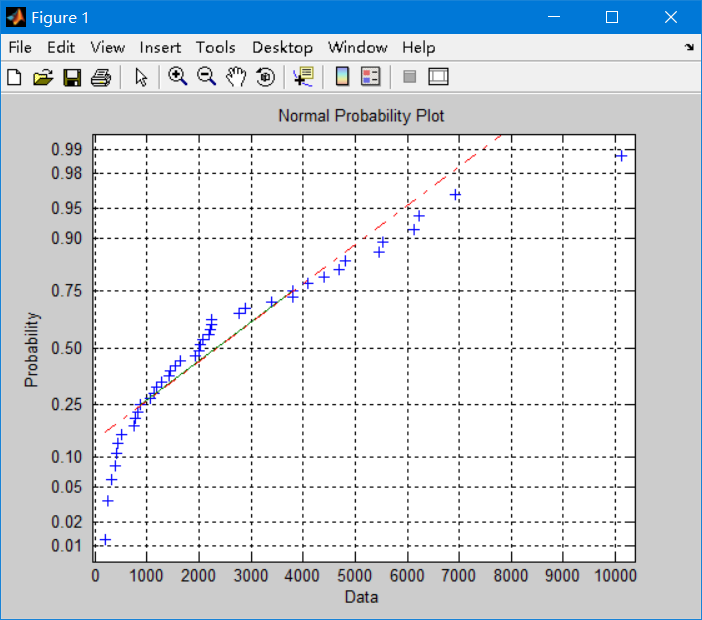从图上可以看出散点并不聚集在直线上，因此流域数据不服从正态分布，但是我们需要检验才能说话，因此我们用jbtest检验
>> [h,P,Jbstat,CV] = jbtest(x,0.05)

h =

1

P =

6.1626e-004

Jbstat =

14.7837

CV =

5.9915

通过检验之后发现，h=1表示在置信水平α=0.05不接受原假设，且P=很小的值，且Jbstat=14.7837大于临界值CV=5.9915，所以不接受原假设，
也就是数据不服从正态分布


展开全文matlab
• 若数据集服从正太分布，则样本点应围绕第一象限的对角线分布。1.2 QQ图以样本的分位数作为横坐标，以按照正太分布计算的相应分位点作为纵坐标，把样本表现为直角坐标系的散点。若服从正太分布，则样本点应该呈一条...
1.图示法1.p-p图以样本的累积频率作为横坐标，以正太分布计算的响应累积概率作为纵坐标，把样本值表现为执教坐标系中的散点。若数据集服从正太分布，则样本点应围绕第一象限的对角线分布。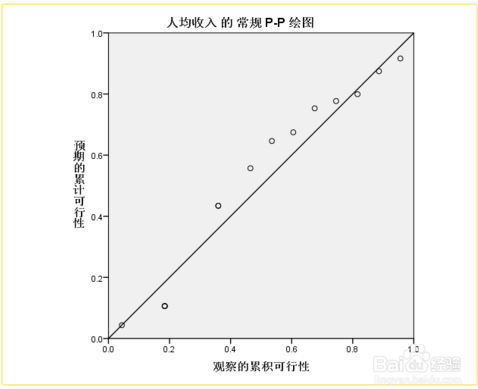1.2 QQ图以样本的分位数作为横坐标，以按照正太分布计算的相应分位点作为纵坐标，把样本表现为直角坐标系的散点。若服从正太分布，则样本点应该呈一条围绕第一象限对角线的直角。https://jingyan.baidu.com/article/48a42057e667bda9242504f5.html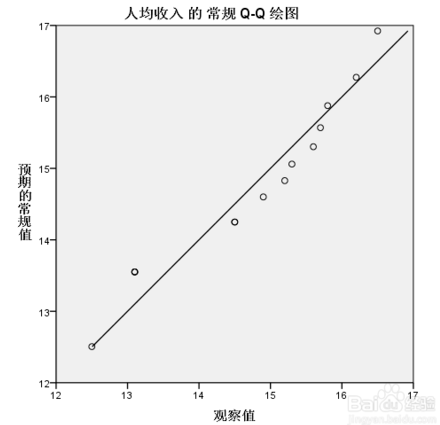1.3直方图（最直观）判断方法：是否以钟形分布，同事可以选择输出正态曲线1.4箱式图判断方法：观测离群值和中位数1.5茎叶图类似于直方图，但实质不同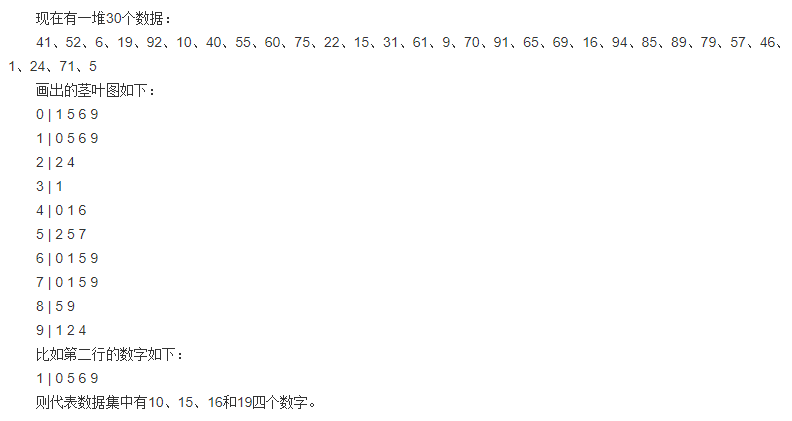2.计算法2.1偏度系数和峰度系数是可以用来衡量数据集的分布形状的系数。偏度系数计算公式：（取值范围通常再-3~3，衡量了数据集的对称程度；0数据集对称，负左侧分散，正右侧分散）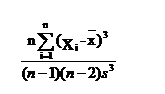峰度系数(负说明数据集较集中，两侧数据集较少。为正则相反；偏度系数和峰度系数都为0，则该数据集服从标准的正态分布)：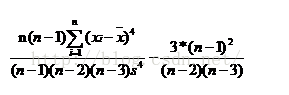https://blog.csdn.net/wzgl__wh/article/details/52080688偏度：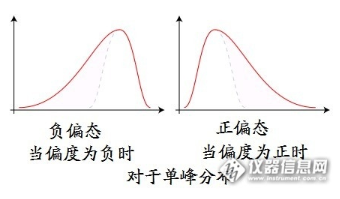峰度：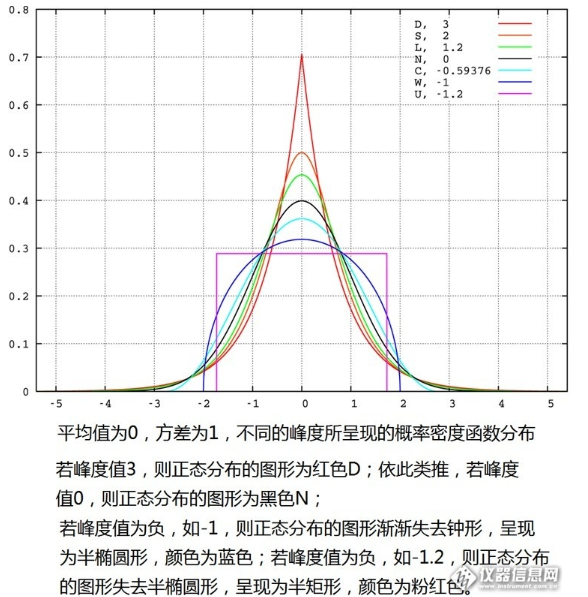http://bbs.instrument.com.cn/topic/5969170/2.2非参数检验方法包括kolmogorov-smirnov检验(D检验)和Shapiro-Wilk（W检验）
展开全文• matlab的Q-Q图能检验数据是否服从正态分布，或者近似正态分布。它认为，如果两个分布都是同一类的，那么他们的分位数之间应该有线性关系。 如果某个分布跟标准正态分布相近，那么它的分位数与正态分布的分位数，应该...matlab qqplot
• 假设检验实战 1.要解决的问题 给出的数据集为人体的体温数据，下载链接为 ... 包括三个数据字段： gender：性别，1为男性，2为女性 ...人体的温度是否服从正态分布? 人体体温中存在...异常数据
• 在样本量比较大时，可根据直方图及对应的正态概率密度曲线的形状大致判断资料是否服从正态分布。 操作：图形-旧对话框-直方图 结果与分析 上图中横坐标为猪崽体重，纵坐标为猪崽频数。可以看出绘制的直方图与...统计学
• （1）检验每组数据是否服从正态分布； 第一组数据利用Matlab编译程序如下： clc; clear; load('c.mat'); c=c';%转置 alpha=0.05;%0.95置信度 % 第一组数据正态分布判断  [mu,sigma]=nor...matlab 数理统计
• 使用K-S检验一个数列是否服从正态分布、两个数列是否服从相同的分布 假设检验的基本思想： 若对总体的某个假设是真实的，那么不利于或者不能支持这一假设的事件A在一次试验中是几乎不可能发生的。如果事件A......